## Programming

### One and Two Input Gates

AND Gate NAND Gate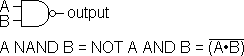Output is TRUE only if both inputs are TRUE.

Output is FALSE only if both inputs are TRUE.

 A    B output F    F F F    T F T    F F T    T T

 A    B output F    F T F    T T T    F T T    T F
OR Gate NOR Gate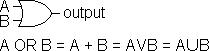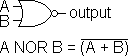Output is TRUE if either input (or both) is TRUE.

Output is FALSE if either input (or both) is TRUE.

 A    B output F    F F F    T T T    F T T    T T

 A    B output F    F T F    T F T    F F T    T F
EXCLUSIVE OR (XOR) Gate EXCLUSIVE NOR (XNOR) Gate
(or Equality Gate)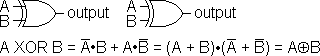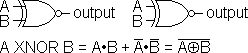Output is TRUE if either (but not both) input is TRUE.

Output is FALSE if either (but not both) input is TRUE.

 A    B output F    F F F    T T T    F T T    T F

 A    B output F    F T F    T F T    F F T    T T
Inverter
(or NOT Function or Compliment Function)A output F T T F

### Three Input Gates

AND Gate NAND Gate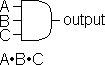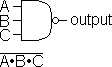Output is TRUE only if all inputs are TRUE.

Output is FALSE only if all inputs are TRUE.

 A    B    C output F    F    F F F    F    T F F    T    F F F    T    T F T    F    F F T    F    T F T    T    F F T    T    T T

 A    B    C output F    F    F T F    F    T T F    T    F T F    T    T T T    F    F T T    F    T T T    T    F T T    T    T F
OR Gate NOR Gate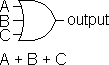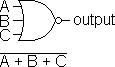Output is TRUE if any input (or all) is TRUE.

Output is FALSE if any input (or all) is TRUE.

 A    B    C output F    F    F F F    F    T T F    T    F T F    T    T T T    F    F T T    F    T T T    T    F T T    T    T T

 A    B    C output F    F    F T F    F    T F F    T    F F F    T    T F T    F    F F T    F    T F T    T    F F T    T    T F

### Boolean Algebra Theorems

 AND theorems 0 · 0 = 0 A · 1 = A 1 · 1 = 1 A · A = A 1 · 0 = 0 A · A = 0 A · 0 = 0 OR theorems 1 + 1 = 1 A + 0 = A 0 + 0 = 0 A + A = A 1 + 0 = 1 A + /A = 1 A + 1 = 1 NOT /0 = 1 /1 = 0 //A = A Commutation A + B = B + A A · B = B · A Absorption A + A · B = A A · (A + B) = A Association A + (B + C) = (A + B) + C A · (B · C) = (A · B) · C Distribution A + B · C = (A + B) · (A + C) A · (B + C) = A · B + A · C DeMorgan's theorems /A + /B = /A · /B /A · /B = /A + /B

### Useful Logical Programming Tools

IF N AND 1 THEN Tests if a number is odd (has its 1 bit set)
N + N AND -2 Always gives the even number of a pair, so if you are calculating an offset into a buffer, this always places you at an even-numbered position.
N = N AND X Keeps a variable within a range X.
So N AND 16383 insures that an integer never exceeds 16383.
N = N AND 127 Strips the high bit from characters such as for sending to a serial port.
N = N OR 128 Sets the high bit (in some cases this signals an attribute such as underlining).
N = N AND 95 Resets bit 6 to 0 so ASCII characters are upper case.
Replaces IF (ASCII) CODE N) > 96 THEN N = N - 32.
N = N OR 32 Makes all letters lower case by setting bit 6.
N = N OR 48 Converts the digits 0 to 9 into their printable ASCII codes (sets bits 5 and 6).
IF X OR Y If either X or Y has a nonzero value ...
X = X XOR 1 Sets a flag variable from 1 or -1 to 0 and back.
Replaces IF X THEN X = 0 ELSE X = 1.
PAGE = PAGE XOR 3 XOR can be used jump back and forth between two values, here between video pages 1 and 2.
The value that toggles between has 1's where changes occur and 0 where they don't, for example to switch between
 3 00000011 and 45 00101101 use 46 00101110
T = T + 1 AND 3 This can be used to maintain a cycle.
Replaces T = T + 1: IF T = 4 THEN T = 0.
T = 1 - T Toggles between 1 and 0.

### Notes

False = 0
True = 1

Positive Logic (or Positive True Logic or High True Logic)
False or 0 = LOW
True or 1 = HIGH

Negative Logic (or Negative True Logic or Low True Logic)
False or 0 = HIGH
True or 1 = LOW

Bit_Logic.vi:  A LabVIEW program that demonstrates bit-wise logic operations.

[  Index  |  Technical Notes Page  ]